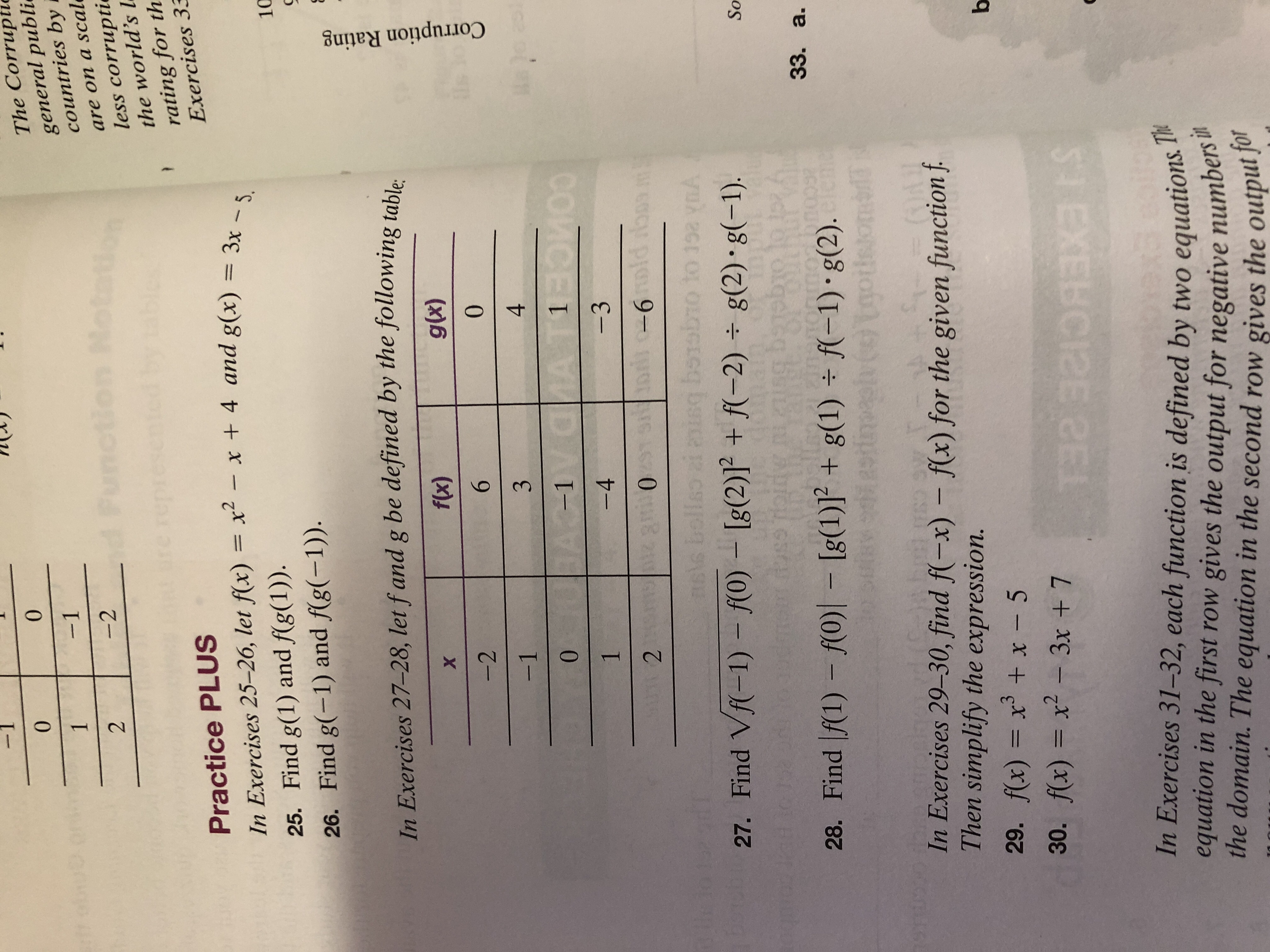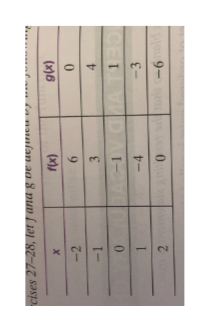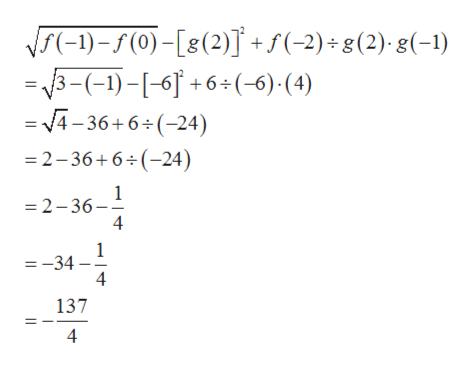00The Corrupticgeneral publicountries by-11totationare on a scalless corruptiethe world's lheFunct-2d2re rerating for thExercises 33Practice PLUSIn Exercises 25-26, let f(x) x- x + 4 and g(x)= 3x - 5.25. Find g(1) and f(g(1))26. Find g(-1) and f(g(-1))10In Exercises 27-28, let f and g be defined by the following tahloflx)glu)X-260-134W!0 A-1 VaIA1001-4-32-6L7 0 8 21OLGCIGG27. Find Vf(-1) - f(0) Ig(2)1 + f(-2) g(2) g(-1)YASoise28. Find f(1) f(0) Ig(1) +g(1) f-1) g(2).33. a.Igo1039TIn Exercises 29-30, find f(-x) f(x) for the given function fThen simplify the expression.(OA29. f(x) x + x - 5b30. f(x) x2-3x + 7STEXEBCIZE CEIn Exercises 31-32, each function is defined by two equations Thequation in the first row gives the output for negative numbers itthe domain. The equation in the second row gives the output forCorruption Rating

Question

Can I get help on #27 and #29help_outlineImage Transcriptionclose0 0 The Corruptic general publi countries by -1 1 totation are on a scal less corruptie the world's l he Funct -2 d 2 re re rating for th Exercises 33 Practice PLUS In Exercises 25-26, let f(x) x- x + 4 and g(x)= 3x - 5. 25. Find g(1) and f(g(1)) 26. Find g(-1) and f(g(-1)) 10 In Exercises 27-28, let f and g be defined by the following tahlo flx) glu) X -2 6 0 -1 3 4 W! 0 A-1 VaIA 100 1 -4 -3 2 -6 L7 0 8 21 OLGCIGG 27. Find Vf(-1) - f(0) Ig(2)1 + f(-2) g(2) g(-1) YA So ise 28. Find f(1) f(0) Ig(1) +g(1) f-1) g(2). 33. a. Igo 10 39T In Exercises 29-30, find f(-x) f(x) for the given function f Then simplify the expression. (OA 29. f(x) x + x - 5 b 30. f(x) x2-3x + 7 STEXEBCIZE CE In Exercises 31-32, each function is defined by two equations Th equation in the first row gives the output for negative numbers it the domain. The equation in the second row gives the output for Corruption Rating fullscreen
Step 1

27). Given,help_outlineImage Transcriptionclose9- 0mto -3 -4 CET D AIVE -1 9 -2 X (x)6 (x) uataea da naalan ag 8 pun (1a) '87-L8e fullscreen
Step 2

Therefore,help_outlineImage Transcriptionclosef(-1)-5(0)-[8(2)]+s(-2)g(2) g(-1) =3-(-1)-[-6+6+(-6) (4) = V4-36+6 (-24) 2-36+6 (-24) 1 2-36 =-34 4 137 4 fullscreen
Step 3

29). Give...

Want to see the full answer?

See Solution

Want to see this answer and more?

Our solutions are written by experts, many with advanced degrees, and available 24/7

See Solution
Tagged in

Equations and In-equations